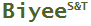Tweet

# Binomial Confidence Interval

## and its application in reliability tests

(last updated on 2019-11-12)

Binomial confidence intervals are used when the data are dichotomous (e.g. 0 or 1, yes or no, success or failure). A binomial confidence interval provides an interval of a certain outcome proportion (e.g. success rate) with a specified confidence level.

Reliability tests are in the category where binomial confidence intervals can be applied. The outcome of each unit of a reliability test is either "success" or "failure".  A typical reliability test assesses a product's reliability (i.e. success rate)  for a specified duration. Each unit either lasts for a specified duration or fails before the duration ends. For the failed units, it does not consider when it fails. The test results are simply two numbers: the sample size n and the number of successful units x. Based on these two numbers, the reliability for any given confidence level, which is a one-sided confidence interval,  can be calculated.

There are two categories of methods to compute binomial confidence intervals - approximation and exact.  The approximations include normal approximation, Wilson score interval, Agresti-Coull interval, Jeffreys interval.  The exact methods are essentially variants the Clopper-Pearson interval. This note focuses on the exact method because it provides us a window to look into the real meaning and complexity of confidence interval.

The word "exact" may give the impression that an exact method provide a crystal clear and simple understanding of how confidence intervals are defined and computed. Crystal clear, maybe.  Simple, probably not.  Thomas Ramsey, PhD provides a succinct description of binomial confidence intervals with rigorous proof of the equations for computing them.  The 4-page paper may not be easily digestible for most users of confidence intervals.  Fortunately, users do not need to understand the proof of the statistical equations applied in their work in most cases. However, a good conceptual understanding is always beneficial.  Dennis P. Wash, PhD wrote an excellent note on exact binomial confidence interval including an intuitive interpretation and examples of computation. Jenő Reiczigel, PhD shows confidence interval construction by test inversion in a paper which provides another perspective on how binomial confidence intervals are derived.  David Harte, PhD has a succinct and practical note on how to compute the intervals using the F-distribution function and how the method is derived.

The following is an effort to expand Wash's note with the hope to help users of different backgrounds enhance their understanding of the concept of confidence interval.

Suppose a study has n trials (e.g. n units under reliability test), x of the n trials are successes (e.g. n - x units failed the test). The lower bound (pLB) of the confidence interval (CI) with a confidence level of 100(1- α)% is obtained by solving the following equation: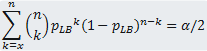(Eq. 1)

The corresponding upper bound (pUB) is obtained by solving the following equation: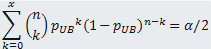(Eq. 2)

Let us use an example to explain why the interval is determined by the above two equations.  Suppose there are 30 trials and 20 are successes, and the desired confidence level is 90% (i.e. α = 0.05).  The lower bound of the CI is the lowest success probability that can be accepted with the current set of data.  Each success probability determines a binomial distribution (Fig. 1 shows two such distributions).  The distribution corresponding to pLB should be such that the current data (i.e. 20 successes out of 30 trials) is barely rejected with α = 0.05.  In other words, the area of the upper tail starting at 20 (the blue tail in Fig. 1 calculated with Eq. 1 ) equals 0.05. In this case, the distribution with p = 0.05 meets the condition, so pLB = 0.50. Similarly, the upper bound (pUB) should be the probability that is barely high enough to reject 20 with the significance level 0.05.  In other words, the area of the lower tail starting at 20 (the red tail  in Fig. 1 calculated with Eq. 2) equals 0.05.  In this case, the distribution with p = 0.81 meets the condition, so pUB = 0.81.  Please note the binomial distribution curves in Fig. 1 comprise discrete points each of which corresponds to an element of Eq. 1 or Eq. 2.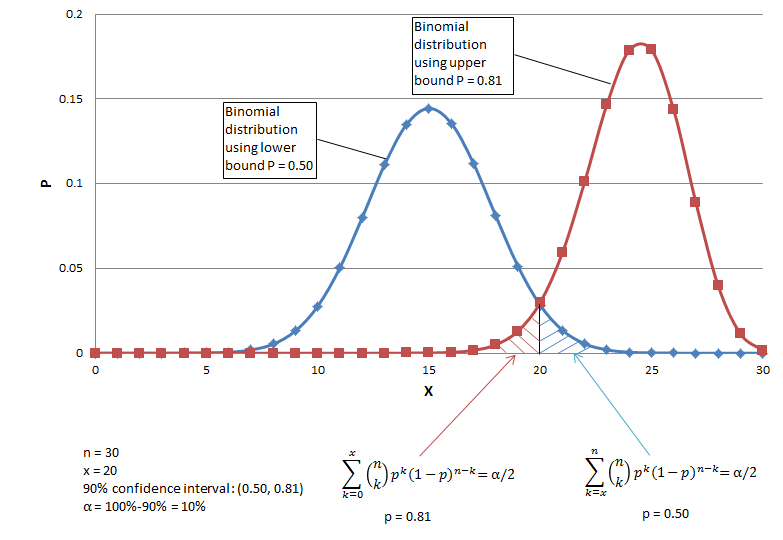Fig. 1 An example of binomial trials and exact confidence interval

The above two equations can be directly used by a program to compute intervals by solving them. A practical way of manually computing binomial confidence intervals is using F-distribution functions. It is also a simple way to for programs with access to an F-distribution function.

The lower bound: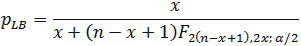(eq. 3)

where F2(n-x+1),2x;α/2 is the F value with 2(n-x+1) numerator degrees of freedom, 2x denominator degrees of freedom and the right tail area of α/2.

The upper bound: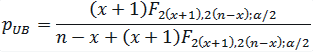(eq.4)

where F2(x+1),2(n-x);α/2 is the F value with 2(x+1) numerator degrees of freedom, 2(n-x) denominator degrees of freedom and the right tail area of α/2.

For reliability tests, only the lower bound is concerned so α/2 should be replaced with α in all the above equations. For example, to compute the lower bound for confidence level 90% (α = 0.1) using the F-distribution function,  F2(n-x+1),2x;0.1 should be used.

Related: